# HBSE 6th Class Maths Solutions Chapter 8 Decimals Ex 8.1

Haryana State Board HBSE 6th Class Maths Solutions Chapter 8 Decimals Ex 8.1 Textbook Exercise Questions and Answers.

## Haryana Board 6th Class Maths Solutions Chapter 8 Decimals Exercise 8.1

Question 1.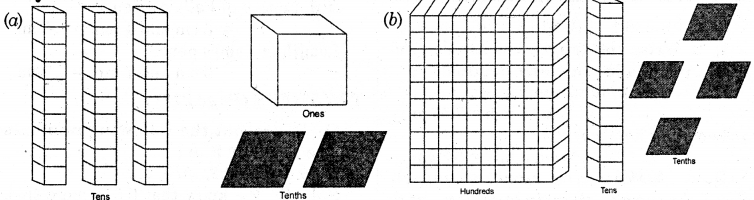Solution: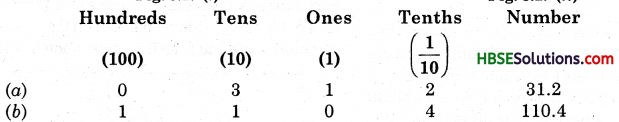Question 2.
Write the following decimals in the (a) 19.4 (6) 0.3 (c) 10.6 (d) 205.9
Solution: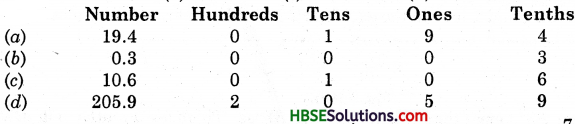Question 3.
Write each of the following as decimals:
(a) 7 tenths
(b) Two tens, 9 tenths
(c) Fourteen point six
(d) One Hundred and 2 ones
(e) Six hundred point eight.
Solution:
(a) 7 tenths = $$\frac{7}{10}$$ = 0.7
(b) Two tens, 9 tenths = 20 + $$\frac{9}{10}$$ = 20.9.
(c) Fourteen point six = 14.6.
(d) Hundred and two ones = 102.0.
(e) Six hundred point eight = 600.8.

Question 4.
Write each of the following as decimals: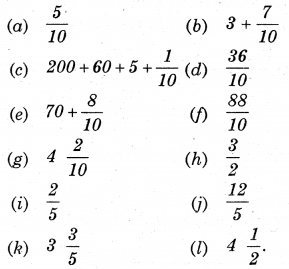Solution: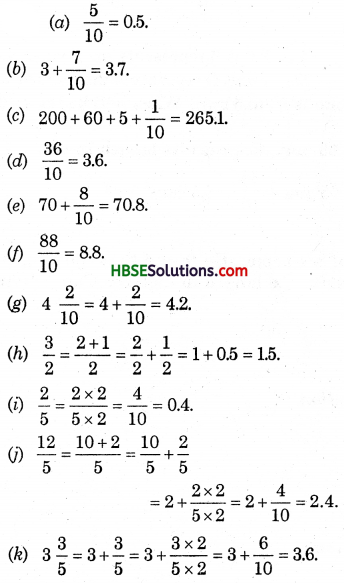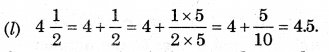Question 5.
Write the following decimals as fractions. Reduce the fractions to their lowest forms : (a) 0.6, (b) 2.5, (c) 1.0, (d) 3.8, (e) 13.7, (f) 21.2, (g) 6.4.
Solution:
(a) 0.6 = $$\frac{6}{10}=\frac{3}{5}$$
(b) 2.5 = $$\frac{25}{10}=\frac{5}{2}$$
(c) 1.0 = 1.
(d) 3.8 = $$\frac{38}{10}=\frac{19}{5}$$
(e) 13.7 = $$\frac{137}{10}$$
(f) 21.2 = $$\frac{212}{10}=\frac{106}{5}$$
(g) 6.4 = $$\frac{64}{10}=\frac{32}{5}$$Question 6.
Express the following as cm using decimals :
(a) 2 mm
(b) 30 mm
(c) 116 mm
(d) 4 cm 2 mm
(e) 162 mm
(f) 83 mm.
Solution:
(a) 2 mm = $$\frac{2}{10}$$ = 0.2 cm.
(b) 30 mm = $$\frac{30}{10}$$ = 3.0 cm
(c) 116 mm = $$\frac{116}{10}$$ = 11.6 cm
(d) 4 cm 2 mm = 4 +$$\frac{2}{10}$$ = 4.2 cm
(e) 162mm = 11 + $$\frac{52}{10}$$ = 11 + 5.2 = 16.2 cm.
(f) 83 mm =$$\frac{83}{10}$$ = 8.3 cm.

Question 7.
Between which two whole numbers on the number line are the given numbers ? Which one is nearer the number ?
(a) 0.8, (b) 5.1, (c) 2.6, (d) 6.4, (e) 9.0, (f) 4.9.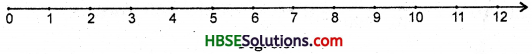Solution:
(a) 0-1, and nearer to the number 1.
(b) 5-6, and nearer to the number 5.
(c) 2-3, and nearer to the number 3.
(d) 6-7, and nearer to the number 6.
(e) 8-10, and nearer to the number 9.
(f) 4-5, and nearer to the number 5.Question 8.
Show the following decimal numbers on the number line :
(a) 0.2,
(b) 1.9,
(c) 1.1,
(d) 2.5.
Solution: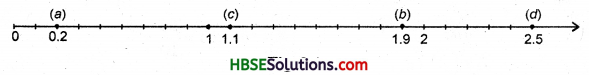Question 9.
Write the decimal number represented by the points A, B, C and D on the given number line :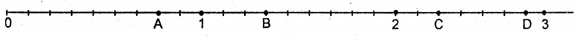Solution:
(i) Point A represents the number 0.8.
(ii) Point B represents the number 1.3
(iii) Point C represents the number 2.2
(iv) Point D represents the number 2.9.Question 10.
(a) The length of Ramesh’s notebook is 9 cm 5 mm. What will be its length in cm ?
(b) The length of a young gram plant is 65 mm. Express its length in cm.
Solution:
(a) 9 cm 5 mm = 9 + $$\frac{5}{10}$$ = 9.5 cm (b) 65 mm = $$\frac{65}{10}$$ = 6.5 cm.